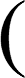Y = a cos x + a cos 2x + a cos 3x + . . . (2)

The motion of the points PP ... is here made harmonic by aid of a series of excentric disks arranged so that for one revolution of the first the other disks complete 2, 3, ... revolutions. They are all driven by one handle. These disks take the place of the templets described before. The distances NG are made equal to the amplitudes a, a, a, ... The drawing-board, moved forward by the turning of the handle, now receives a curve of which (2) is the equation. If all excentrics are turned through a right angle a sine-series can be added up.

It is a remarkable fact that the same machine can be used as a harmonic analyser of a given curve. Let the curve to be analysed be set off along the levers NG so that in the old notation it is

y′ = f(x),

whilst the curves y′ = φ(xξ) are replaced by the excentrics, hence ξ by the angle θ through which the first excentric is turned, so that y′ = cos kθ. But kh = x and nh = π, n being the number of springs s, and π taking the place of c. This makes

 kθ = nπ θ.x.

Hence our instrument draws a curve which gives the integral (1) in the form

 y = 2π ∫π f(x)cosnπ θxdx

as a function of θ. But this integral becomes the coefficient a in the cosine expansion if we make

θn/π = m or θ = mπ/n.

The ordinates of the curve at the values θ = π/n, 2π/n, ... give therefore all coefficients up to m = 80. The curve shows at a glance which and how many of the coefficients are of importance.

The instrument is described in Phil. Mag., vol. xlv., 1898. A number of curves drawn by it are given, and also examples of the analysis of curves for which the coefficients a are known. These indicate that a remarkable accuracy is obtained.

(O. H.)

 For a fuller description of the manner in which a mere addition machine can be used for multiplication and division, and even for the extraction of square roots, see an article by C.V. Boys in Nature, 11th July 1901.